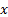/

### Function Description

Returns the probability mass function for a givenfor a (discrete) probability distribution given by DistributionName and with parameter values given in ParamValues (with their order matching the parameter names listed by MnProbDistParamNames). To identify whether a given distribution is continuous (so has a probability density function) or discrete (so has a probability mass function) see MnProbDistIsCts.

Probability distributions currently recognised by the website may be listed using MnRecognisedProbDists.

Contents | Prev | Next